# 前言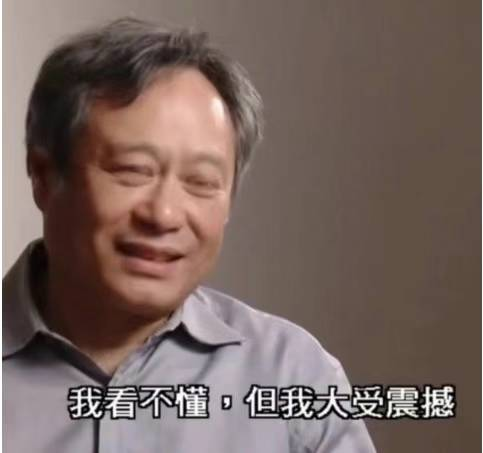100dB是什么概念呢?

20\lg{S} = 100dB
S = {Vpp(noise)\over{Vpp(Signal)}} = 10^5 = 10万倍

# 锁定放大器(Lock-in Amplifier-LIA)基本原理

## 数学原理

1. 我有一个和待测信号同频率的正弦波，称为参考信号
2. 将参考信号与含有广谱噪声的待测正弦波相乘（使用乘法器）
3. 乘法器的输出得到一个含有直流分量的2倍频正弦波（相对于参考信号2倍频）
4. 这个2倍频信号的直流分量与待测正弦波幅度成正比，与两个正弦波相移有关。
5. 如果用低通滤波器滤除这个2倍频，只保留直流分量，就可以得到一个正比于待测信号幅度的直流量

A_{m1}\sin{(w_1t+\phi_1)} * A_{m2}\sin{(w_2t+\phi_2)} = \\
0.5A_{m1}A_{m2}\cos{((w_1-w_2)t+\phi_1-\phi_2)} - \\
0.5A_{m1}A_{m2}\sin{((w_1+w_2)t+\phi_1+\phi_2)}

A_{m1}\sin{(wt+\phi_1)}*A_{m2}\sin{(wt+\phi_2)} = \\
0.5A_{m1}*A_{m2}\cos{(\phi_1-\phi_2)} - \\
0.5A_{m1}*A_{m2}\sin{(2wt+\phi_1+\phi_2)}

A_{m1}\sin{(wt+\phi)}*A_{m2}\sin{(wt+\phi)} = \\
0.5A_{m1}*A_{m2} - 0.5A_{m1}*A_{m2}\sin{(2wt+2\phi)}

A_{m2}\sin(wt+\phi)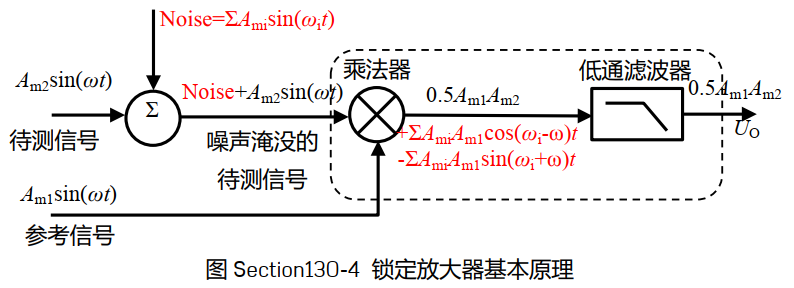## 额外的优点

0.5A_{m1}A_{m2}\cos((w_1-w_2)t+\phi_1-\phi_2)

• 低通滤波器不理想。与参考信号频率相差非常小（甚至同频率）的噪声所产生的交流成分的频率很低。
• 而低通滤波器很难做到极低的截止频率，又同时又具有极低的群延迟 (更正:不关群延迟的事，我概念记歪了，这里应该考察低通滤波器的输出建立时间，感谢小白指正)。这会导致低通滤波器的输出会有微弱起伏。

but，这种方法非常麻烦。

## Solution2:双相位法

设待测信号为： A_{m2}\sin(wt+\phi_2)  \\

U_{OA} = 0.5A_{m1}A_{m2}\cos(\phi_{1A}-\phi_{2}) \\
U_{OB} = 0.5A_{m1}A_{m2}\cos(\phi_{1A}-\phi_{2}+{\pi\over 2}) = 0.5A_{m1}A_{m2}\sin(\phi_{1A}-\phi_2)

U_O = \sqrt{U^2_{OA} + U^2_{OB}}=0.5A_{m1}A_{m2}\sqrt{sin^2(\phi_{1A}-\phi_2)+\cos^2(\phi_{1A}-\phi_2)} = 0.5A_{m1}A_{m2}

\tan(\phi_{1A}-\phi_2) = {\sin(\phi_{1A}-\phi_2)\over\cos(\phi_{1A}-\phi_2)}={U_{OB}\over U_{OA}} \\
\phi_{1A}-\phi_2=tan^{-1}{U_{OA}\over U_{OB}}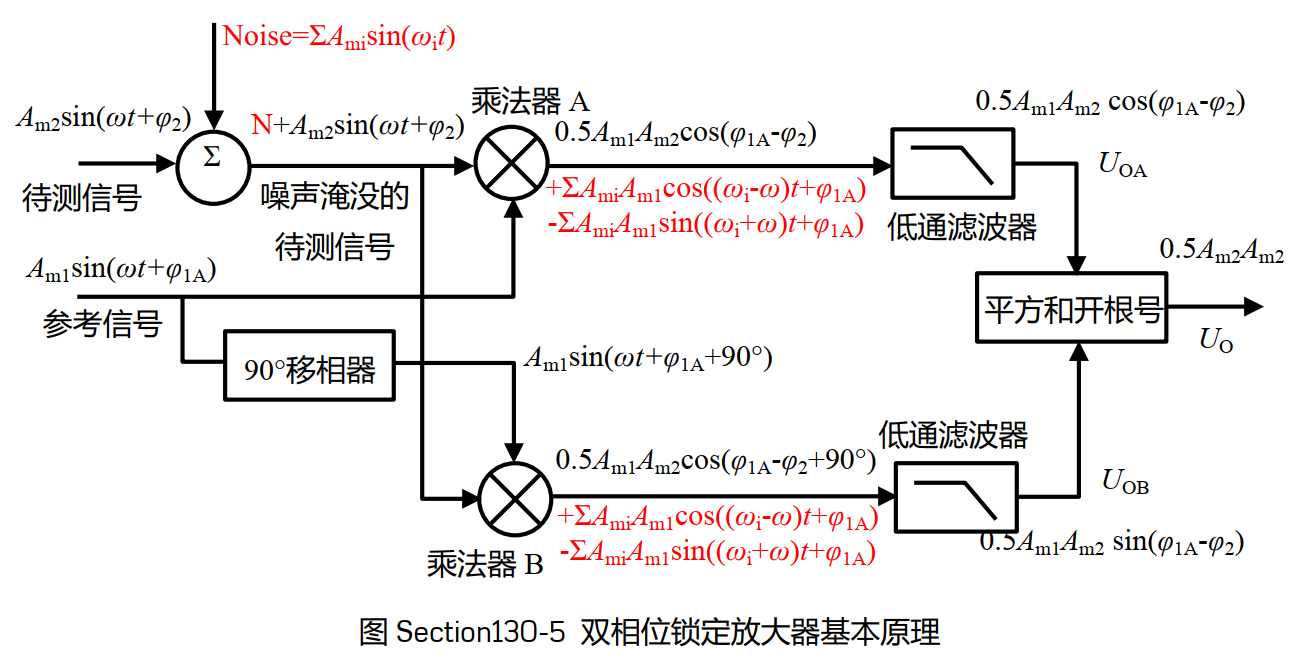# 从理论到实践——方波作为参考信号

1. 使用占空比为50%的正负方波, 其幅度为A_{m1},角频率w，相移为 \phi_1

U_{REF}(t) = \left\{ \begin{array} {c} A_{m1}; {\phi_1 \over w}\leq MOD(t,T={2\pi\over w})<{\phi_1 \over w}+{\pi \over w} \\ -A_{m1}; MOD(t,T={2\pi\over w}) < {\phi_1 \over w}; and\,MOD(t,T={2\pi\over w}) \geq {\phi_1 \over w}+{\pi \over w} \end{array} \right.

1. 此方波与频率相同，相位相同的正弦波相乘，会出现如下图所示的波形。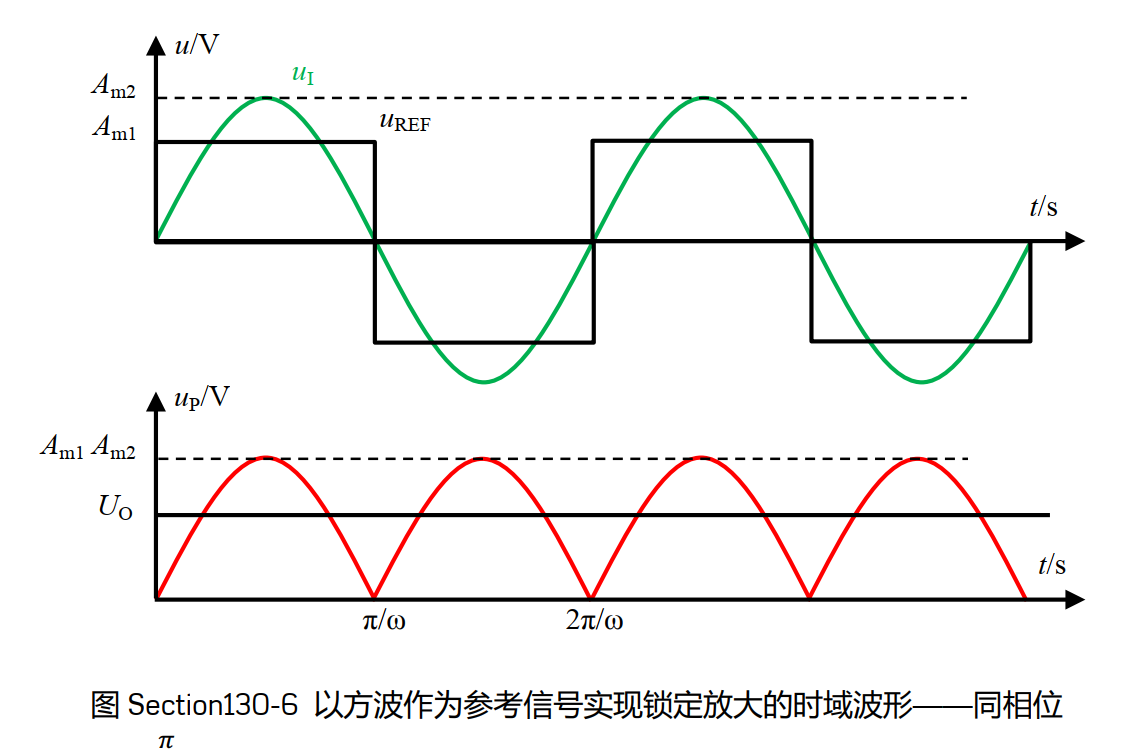U_o={1\over {\pi \over w}} \int_0^{\pi \over w} {A_{m1}*A_{m2}\sin(wt\,dt)} = {2 \over \pi}A_{m1}*A_{m2} \approx 0.6366A_{m1}*A_{m2}

1. 当以待测波形0度相移，而参考波形具有 \phi_1 相移，可以得到Section130-7所示如下的时域波形: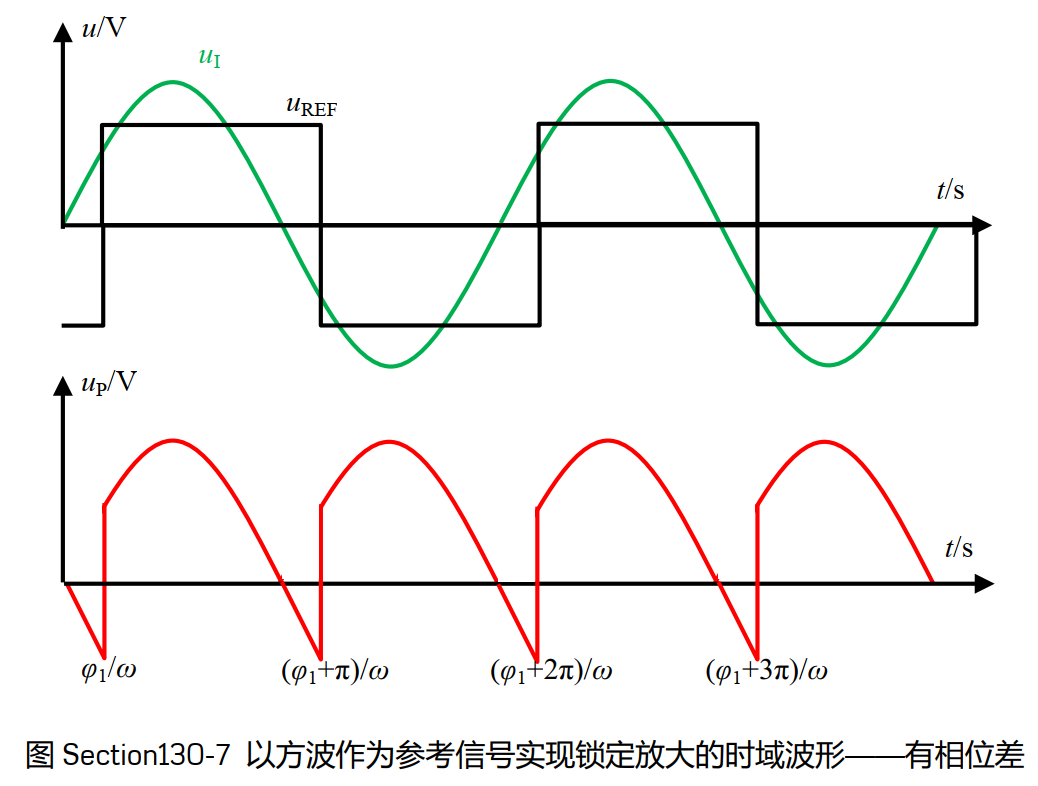U_o = {1\over \pi} \int_{\phi_1}^{\phi_1+\pi} A_{m1}*A_{m2}\sin(wt)\,d(wt) = {2\over \pi}A_{m1}*A_{m2}\cos \phi_1 \approx 0.6366A_{m1}*A_{m2}\cos \phi_1

4.当待测信号与参考信号频率不同时，可证明乘法器输出是一个 正负对称信号，经过低通滤波之后输出一定为0.

• 1.不用再用贵上天的乘法器了！我们可以使用 模拟开关 和反相器来实现锁定放大，结构如下: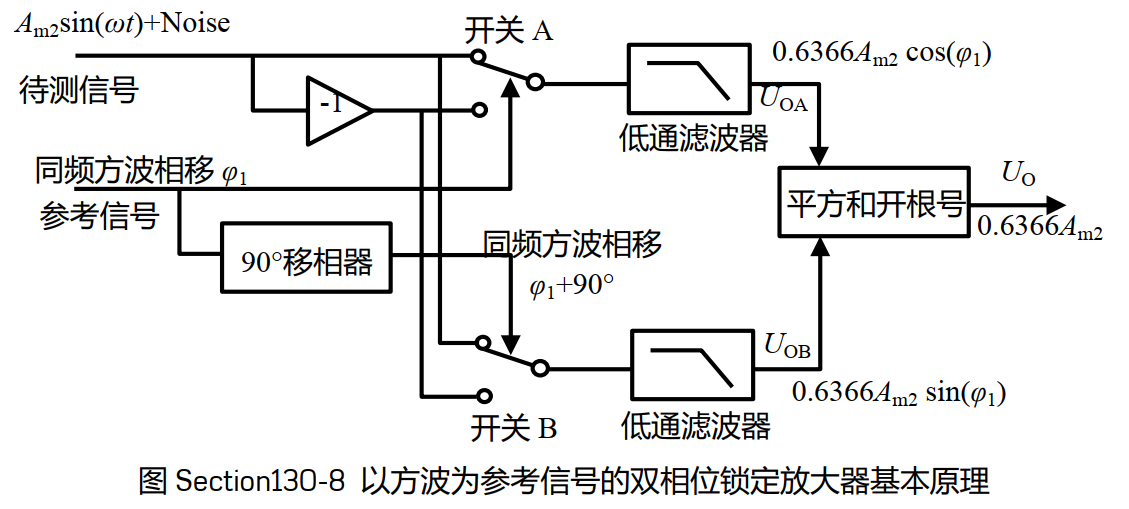• 2.对方波信号进行90度移相还不简单？上图中方波用于控制开关的导向，因此只需要是数字电平的0&1即可。这样就完全可以使用数字电路来控制。用数字电路产生90°相移，可以用单片机、FPGA、触发器等完成，方法很多。

# LIA的用法？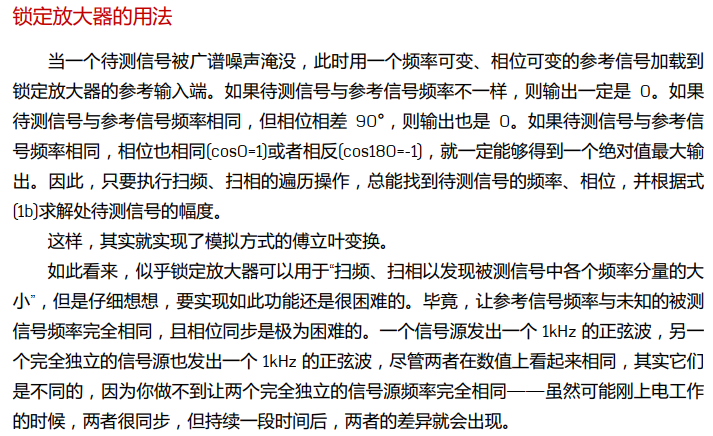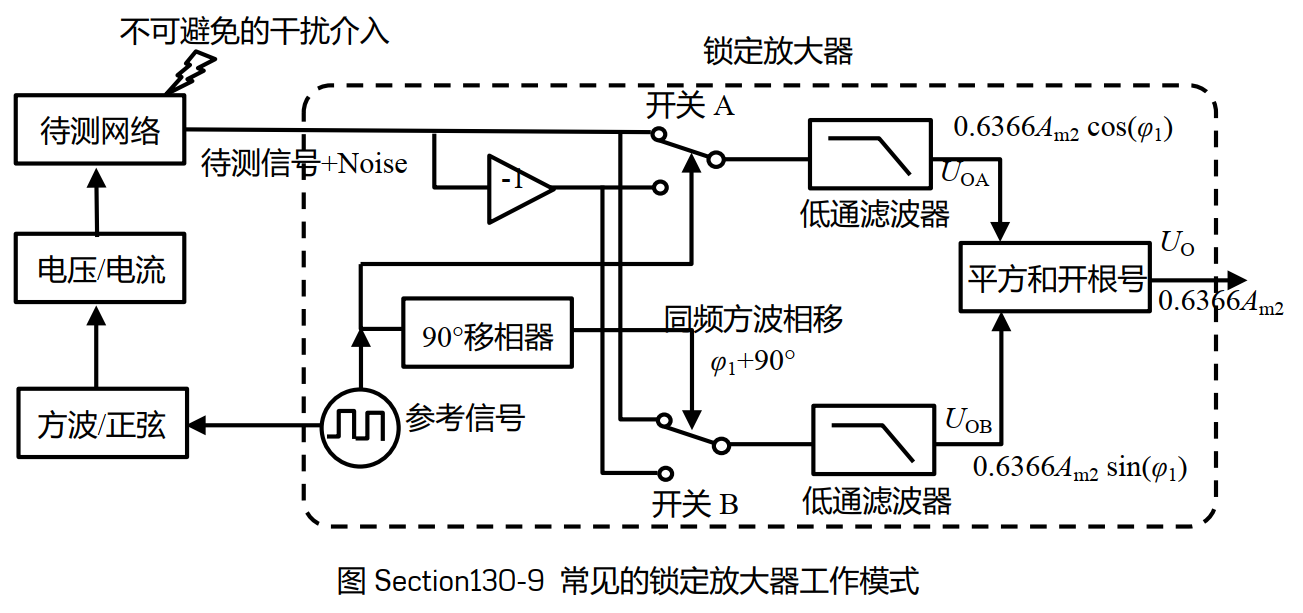# 使用比较器+模拟开关实现LIA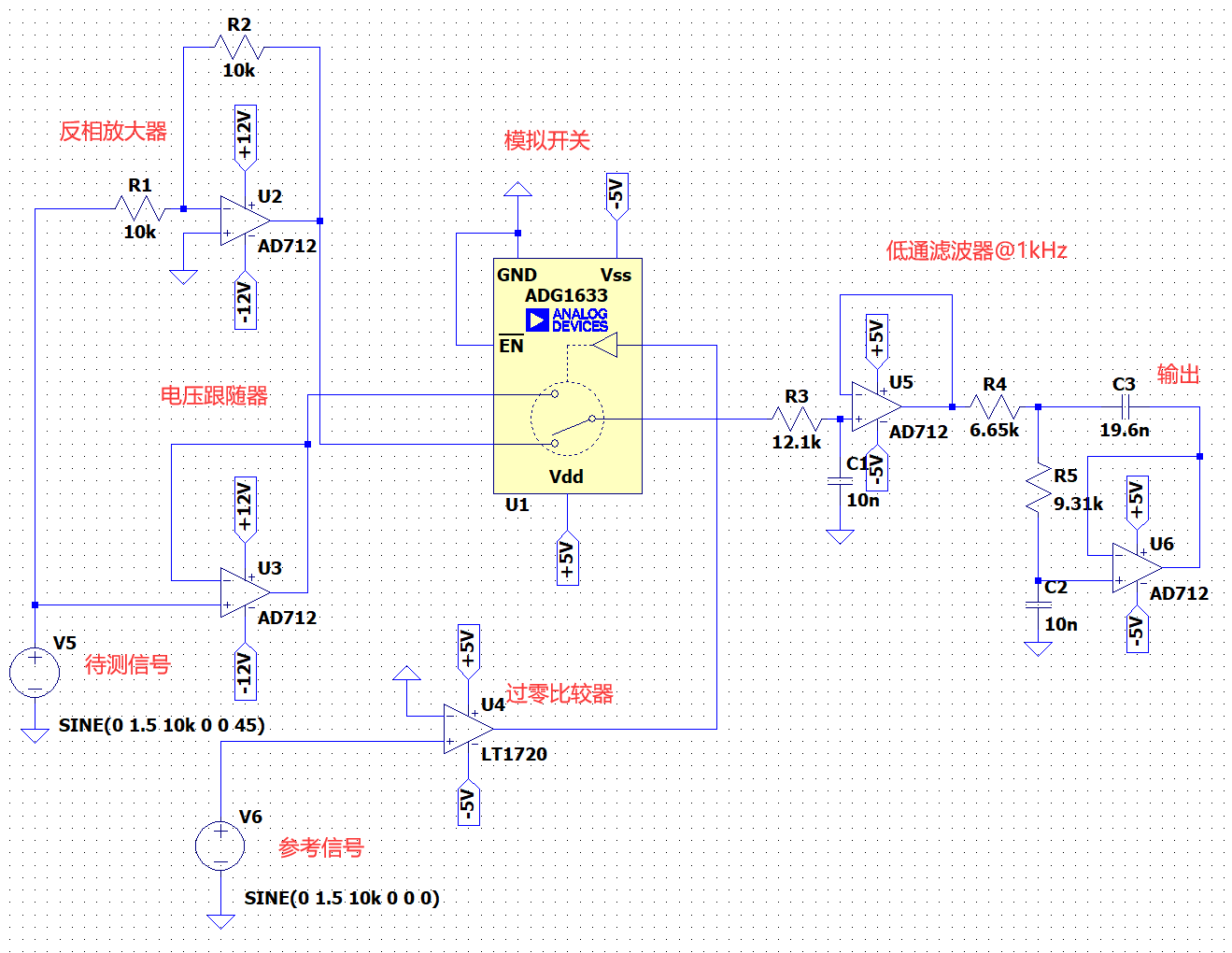0.6366*1.5*cos(\phi) \\
when \phi = 0\degree, \,V_{DC}=0.9549V \\
when \phi = 45\degree, \,V_{DC}=0.6751V \\
when \phi = 90\degree, \,V_{DC}=0.0V \\
when \phi = 180\degree, \,V_{DC}=-0.9549V \\

• 180度相移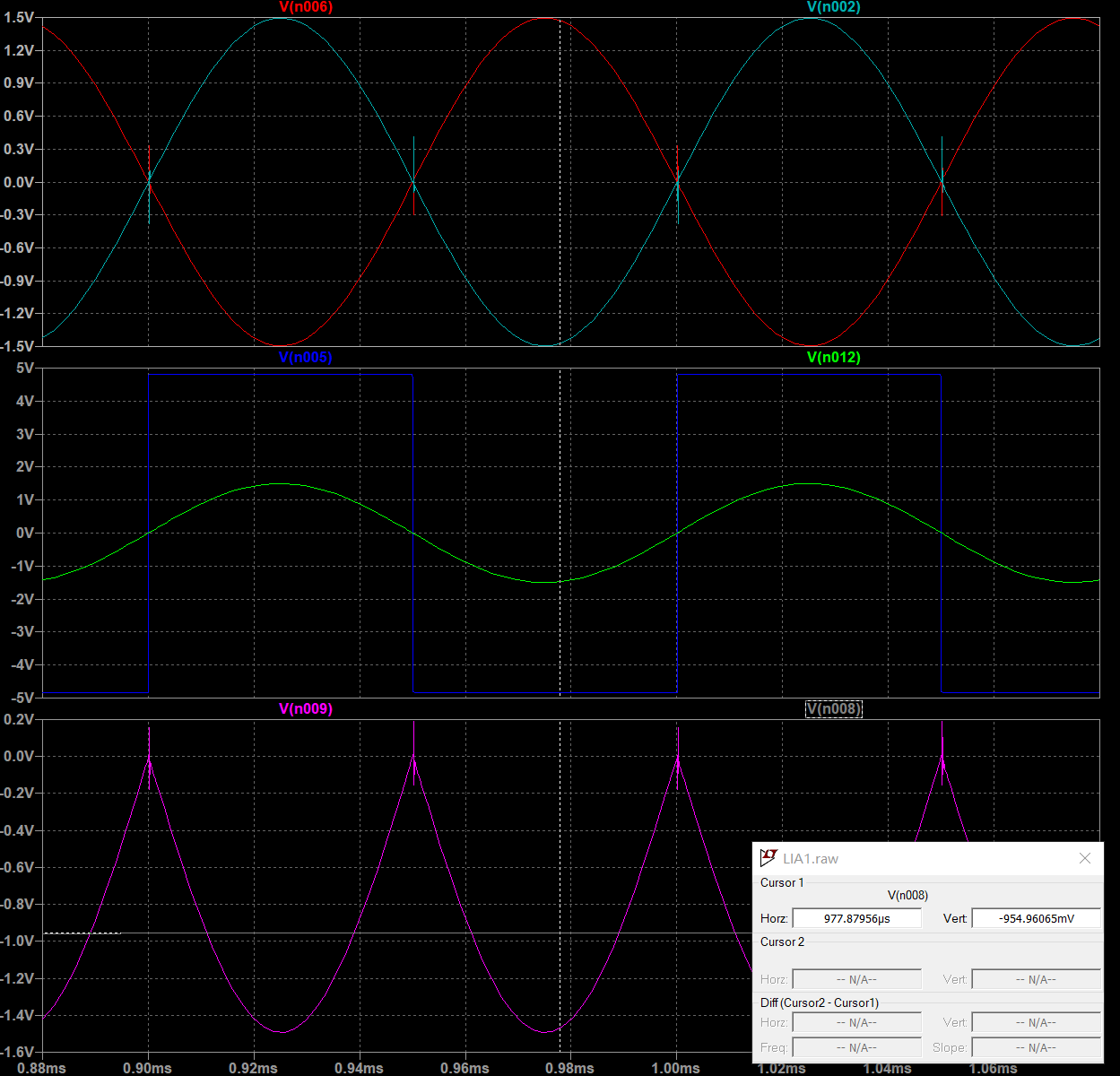• 90度相移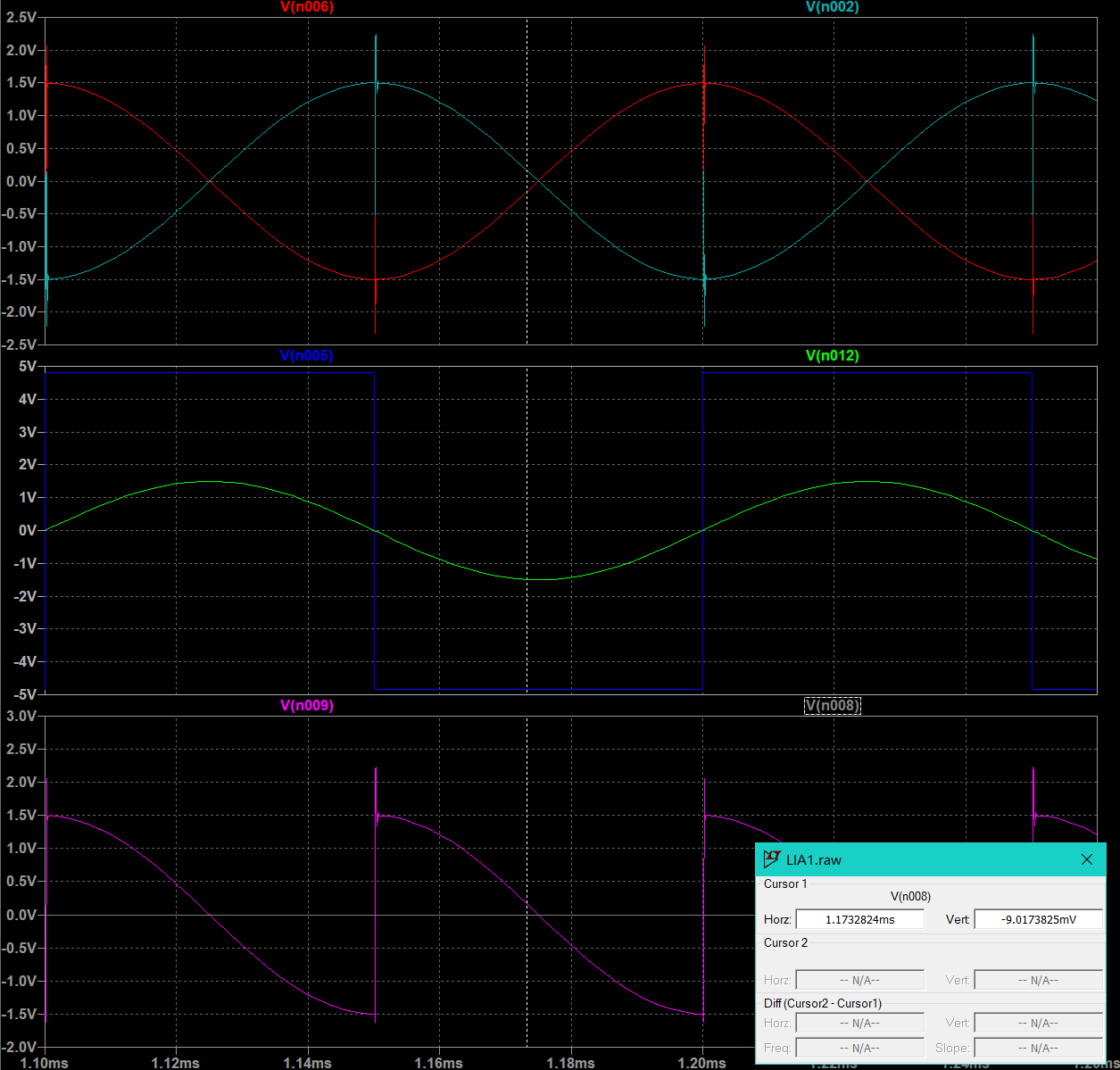• 45度相移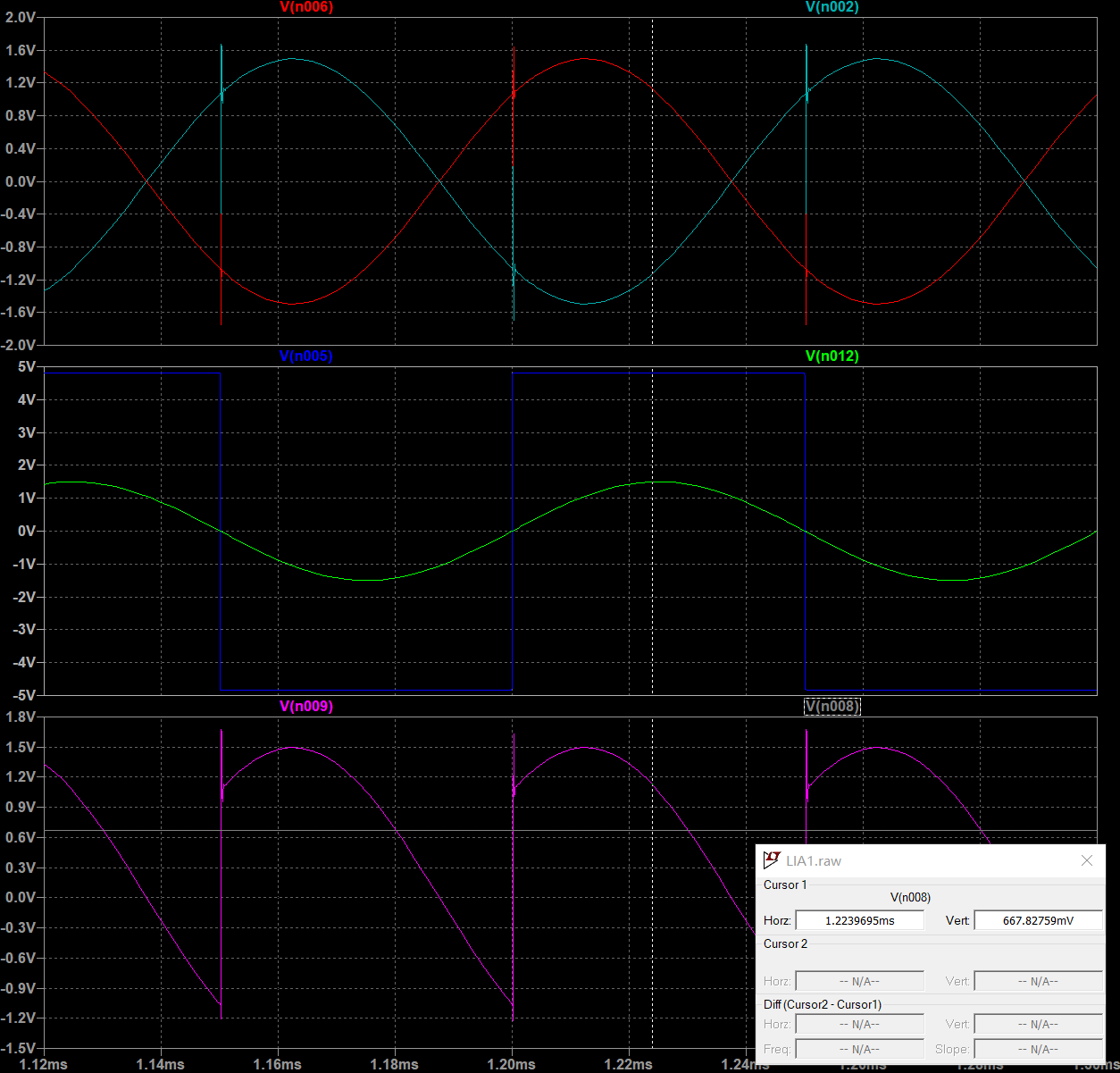• 0度相移（同相）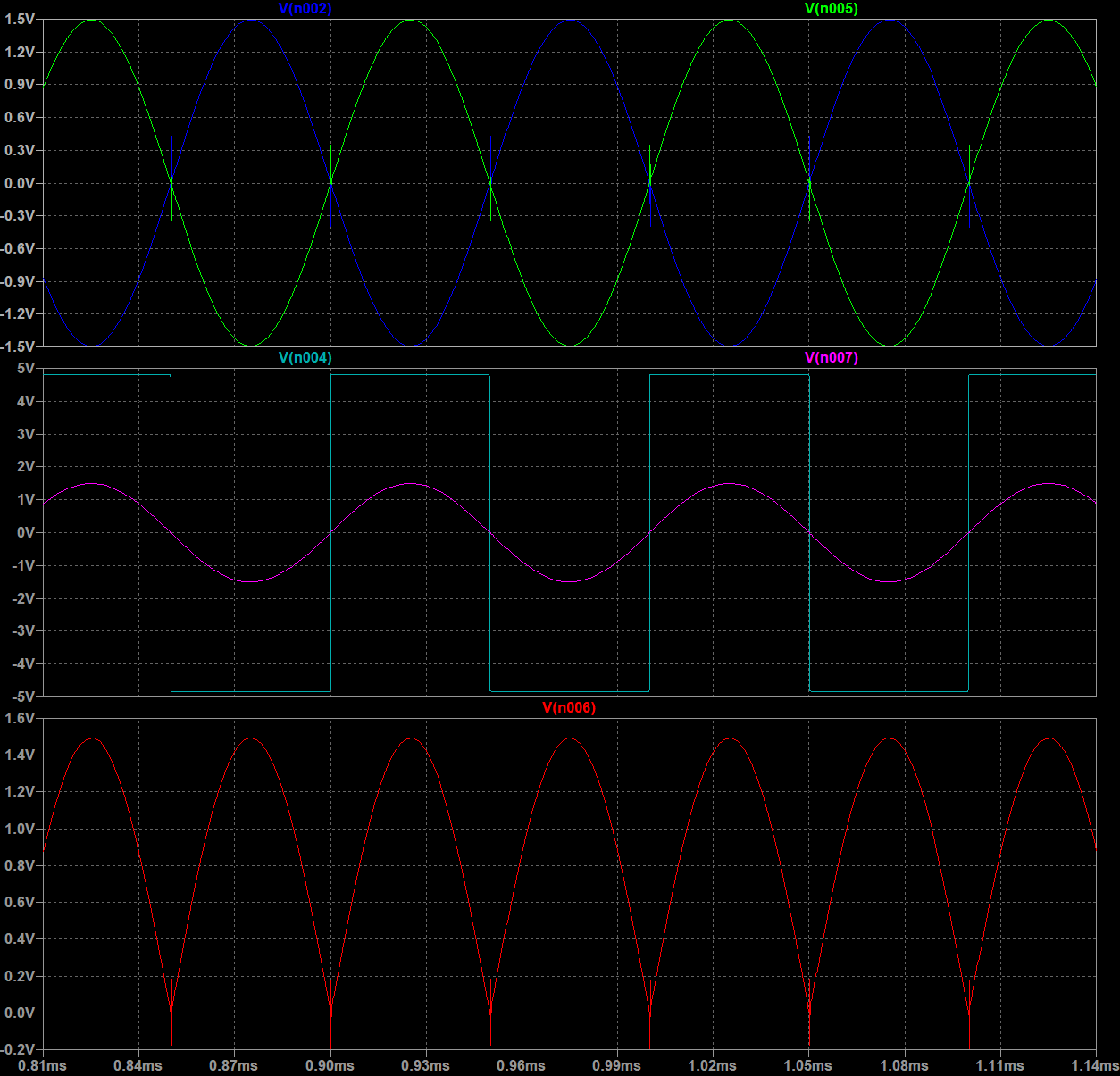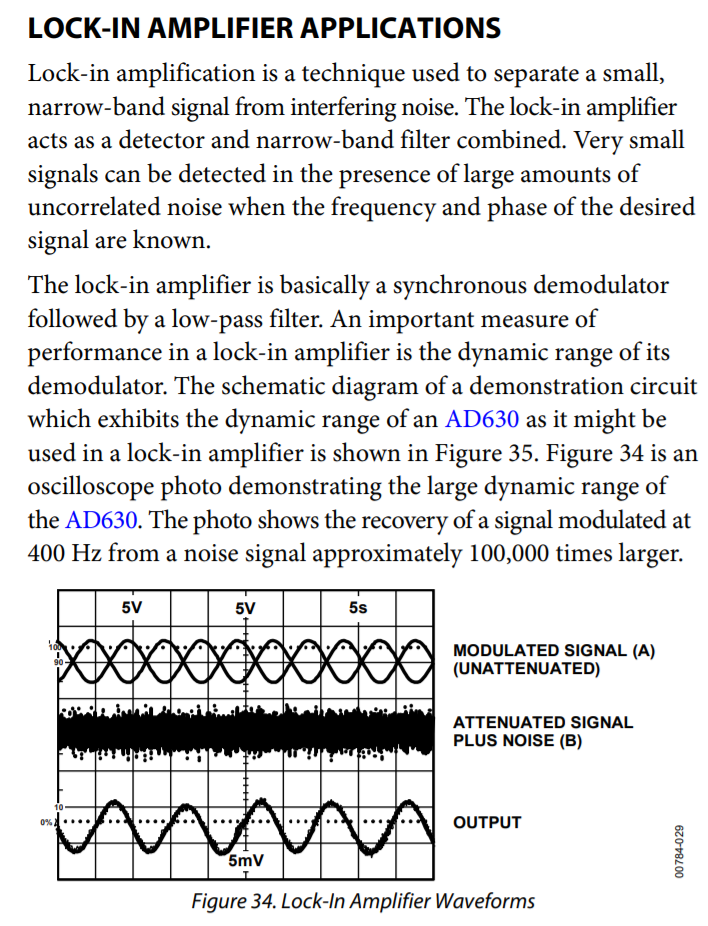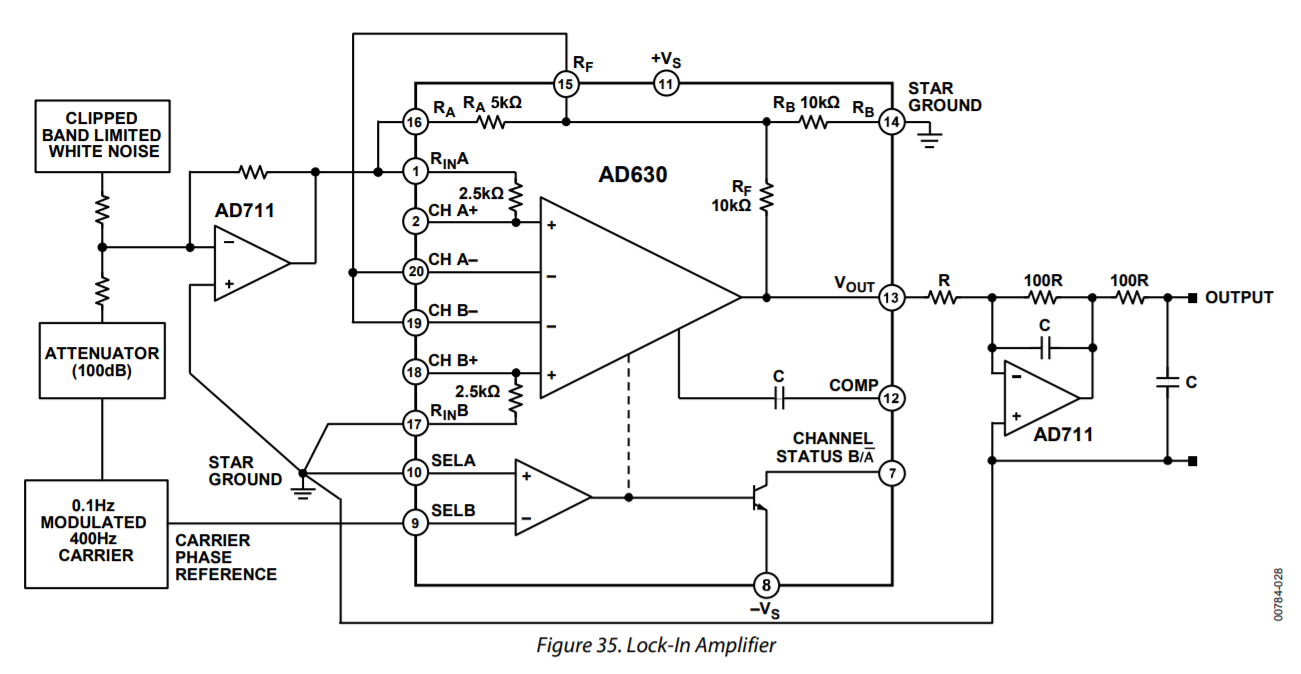• 输出经过一个反相放大器之后再经过一RC低通滤波器，得到解调之后的输出信号。

# 试一试呗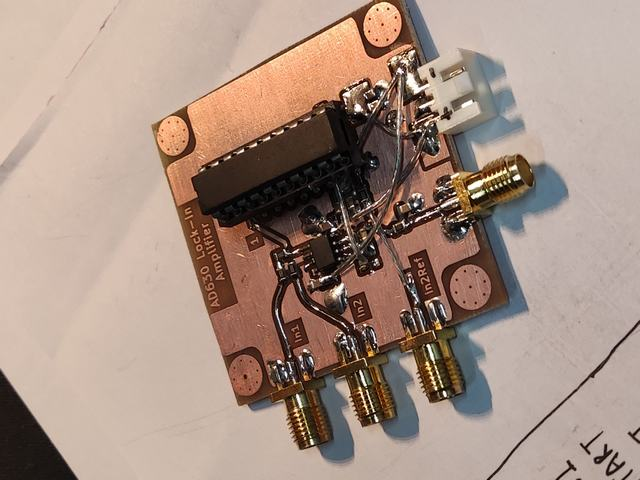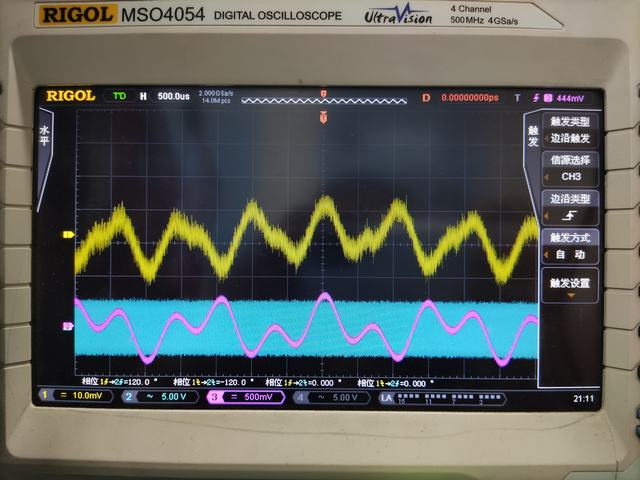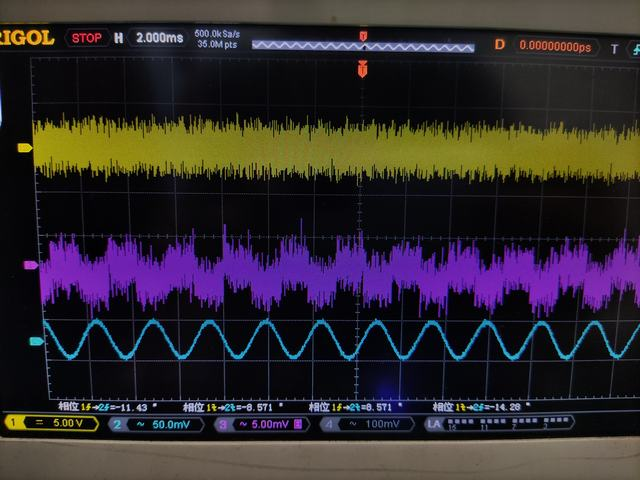# Plan

### 这篇文章有 2 个评论

1.第 虾球页

我随看不懂但我大受震撼.jpg

2.第 Floyd-Fish页

消灭零评论惨案。